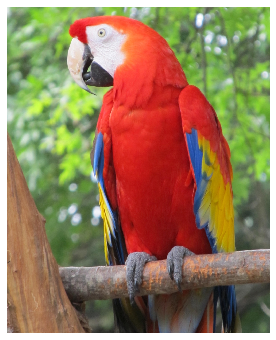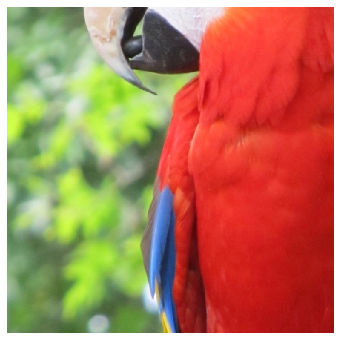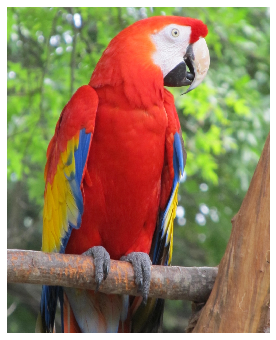# How to save and load parameters of an augmentation pipeline¶

Reproducibility is very important in deep learning. Data scientists and machine learning engineers need a way to save all parameters of deep learning pipelines such as model, optimizer, input datasets, and augmentation parameters and to be able to recreate the same pipeline using that data. Albumentations has built-in functionality to serialize the augmentation parameters and save them. Then you can use those parameters to recreate an augmentation pipeline.

### Import the required libraries¶

import random

import numpy as np
import cv2
import matplotlib.pyplot as plt
import albumentations as A


### Define the visualization function¶

def visualize(image):
plt.figure(figsize=(6, 6))
plt.axis('off')
plt.imshow(image)


### Load an image from the disk¶

image = cv2.imread('images/parrot.jpg')
image = cv2.cvtColor(image, cv2.COLOR_BGR2RGB)
visualize(image)### Define an augmentation pipeline that we want to serialize¶

transform = A.Compose([
A.RandomCrop(768, 768),
A.OneOf([
A.RGBShift(),
A.HueSaturationValue()
]),
])


We can pass an instance of augmentation to the print function, and it will print the string representation of it.

print(transform)

Compose([
RandomCrop(always_apply=False, p=1.0, height=768, width=768),
OneOf([
RGBShift(always_apply=False, p=0.5, r_shift_limit=(-20, 20), g_shift_limit=(-20, 20), b_shift_limit=(-20, 20)),
HueSaturationValue(always_apply=False, p=0.5, hue_shift_limit=(-20, 20), sat_shift_limit=(-30, 30), val_shift_limit=(-20, 20)),
], p=0.5),



Next, we will fix the random seed to make augmentation reproducible for visualization purposes and augment an example image.

random.seed(42)
transformed = transform(image=image)
visualize(transformed['image'])## Serializing an augmentation pipeline to a JSON or YAML file¶

To save the serialized representation of an augmentation pipeline to a JSON file, use the save function from Albumentations.

A.save(transform, '/tmp/transform.json')


To load a serialized representation from a JSON file, use the load function from Albumentations.

loaded_transform = A.load('/tmp/transform.json')

print(loaded_transform)

Compose([
RandomCrop(always_apply=False, p=1.0, height=768, width=768),
OneOf([
RGBShift(always_apply=False, p=0.5, r_shift_limit=(-20, 20), g_shift_limit=(-20, 20), b_shift_limit=(-20, 20)),
HueSaturationValue(always_apply=False, p=0.5, hue_shift_limit=(-20, 20), sat_shift_limit=(-30, 30), val_shift_limit=(-20, 20)),
], p=0.5),



Next, we will use the same random seed as before and apply the loaded augmentation pipeline to the same image.

random.seed(42)assert np.array_equal(transformed['image'], transformed_from_loaded_transform['image'])


As you see, it produced the same result.

#### Using YAML insted of JSON¶

You can also use YAML instead of JSON for serializing and deserializing of augmentation pipelines. To do that add the data_format='yaml' argument to the save and load functions.

A.save(transform, '/tmp/transform.yml', data_format='yaml')

print(loaded_transform)

Compose([
RandomCrop(always_apply=False, p=1.0, height=768, width=768),
OneOf([
RGBShift(always_apply=False, p=0.5, r_shift_limit=(-20, 20), g_shift_limit=(-20, 20), b_shift_limit=(-20, 20)),
HueSaturationValue(always_apply=False, p=0.5, hue_shift_limit=(-20, 20), sat_shift_limit=(-30, 30), val_shift_limit=(-20, 20)),
], p=0.5),



## Serializing an augmentation pipeline to a Python dictionary¶

If you need more control over a serialized pipeline, e.g., you want to save a serialized version to a database or send it to a server you can use the to_dict and from_dict functions. to_dict returns a Python dictionary that describes a pipeline. The dictionary will contain only primitive data types such as dictionaries, lists, strings, integers, and floats. To construct a pipeline from a dictionary, you need to call from_dict.

transform_dict = A.to_dict(transform)

print(loaded_transform)

Compose([
RandomCrop(always_apply=False, p=1.0, height=768, width=768),
OneOf([
RGBShift(always_apply=False, p=0.5, r_shift_limit=(-20, 20), g_shift_limit=(-20, 20), b_shift_limit=(-20, 20)),
HueSaturationValue(always_apply=False, p=0.5, hue_shift_limit=(-20, 20), sat_shift_limit=(-30, 30), val_shift_limit=(-20, 20)),
], p=0.5),



## Serializing and deserializing Lambda transforms¶

Lambda transforms use custom transformation functions provided by a user. For those types of transforms, Albumentations saves only the name and the position in the augmentation pipeline. To deserialize an augmentation pipeline with Lambda transforms, you need to manually provide all Lambda transform instances using the lambda_transforms argument.

Let's define a function that we will use to transform an image.

def hflip_image(image, **kwargs):
return cv2.flip(image, 1)


Next, we create a Lambda transform that will apply the hflip_image function to input images. Note that to make the transform serializable, you need to pass the name argument.

hflip = A.Lambda(name='hflip_image', image=hflip_image, p=0.5)
transform = A.Compose([hflip])
print(transform)

Compose([



To check that transform is working, we will apply to an image.

random.seed(7)
transformed = transform(image=image)
visualize(transformed['image'])To serialize a pipeline with a Lambda transform, use the save function as before.

A.save(transform, '/tmp/lambda_transform.json')


To deserialize a pipeline that contains Lambda transforms, you need to pass names and instances of all Lambda transforms in a pipeline through the lambda_transforms argument.

loaded_transform = A.load('/tmp/lambda_transform.json', lambda_transforms={'hflip_image': hflip})

print(loaded_transform)

Compose([



Verify that the deserialized pipeline produces the same output.

random.seed(7)

To serialize and deserialize Lambda transforms to and from dictionaries use to_dict and from_dict.
transform_dict = A.to_dict(transform)

{'__version__': '0.4.5', 'transform': {'__class_fullname__': 'albumentations.core.composition.Compose', 'p': 1.0, 'transforms': [{'__type__': 'Lambda', '__name__': 'hflip_image'}], 'bbox_params': None, 'keypoint_params': None, 'additional_targets': {}}}


loaded_transform = A.from_dict(transform_dict, lambda_transforms={'hflip_image': hflip})